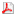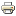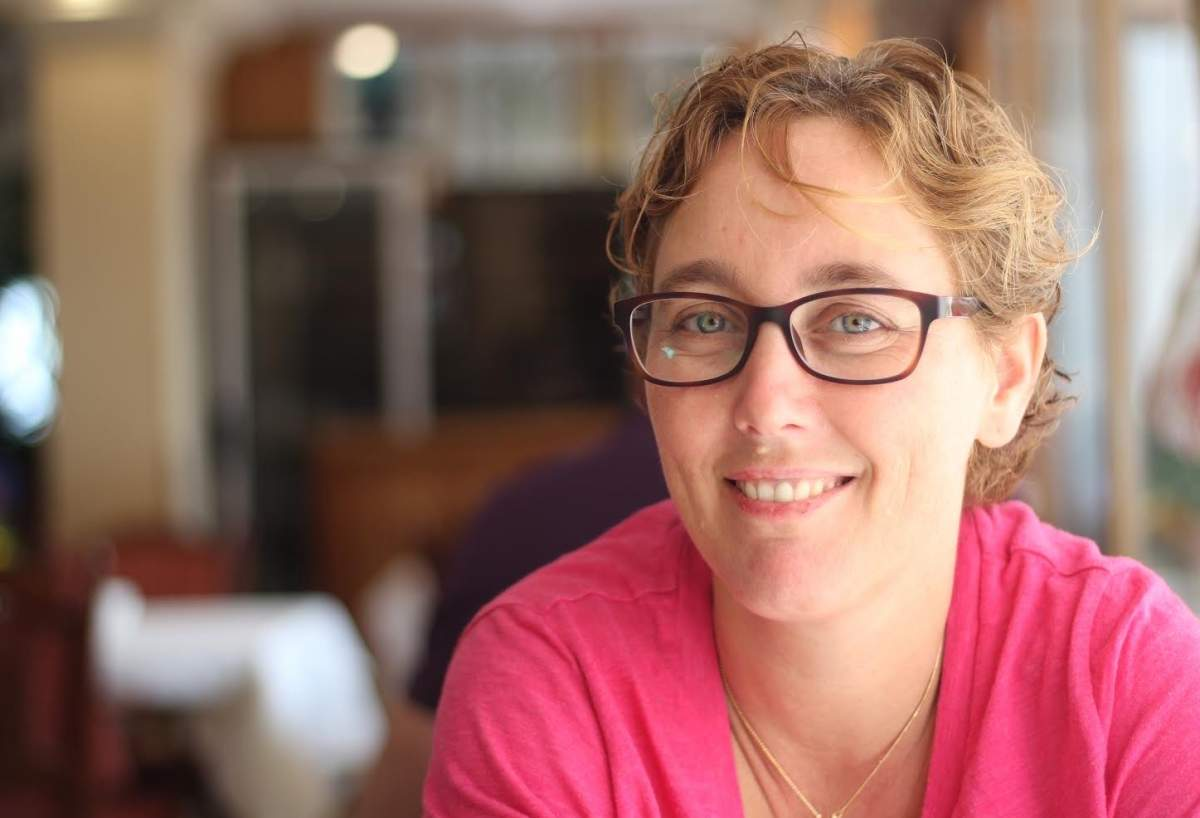MUNI Seminar series - Irit Dinur - Expanders in higher dimensions#### Mathematics, Physics & Computer Science Seminar Series

October 26, 2022 from 4:30 PM at Governor's Palace, Moravské náměstí 1a, Baroque Hall

#### Irit Dinur

Expanders in higher dimensions

Abstract:

Expander graphs have been studied in many areas of mathematics and in computer science with versatile applications, including coding theory, networking, computational complexity and geometry.

High-dimensional expanders are a generalization that has been studied in recent years and their promise is beginning to bear fruit. In the talk, I will survey some powerful local to global properties of high-dimensional expanders, and describe several interesting applications, ranging from convergence of random walks to construction of locally testable codes that prove the c3 conjecture (namely, codes with constant rate, constant distance, and constant locality).

==================================
The PCP theorem

The PCP theorem says that any mathematical proof can be written in a special "PCP" format such that it can be verified, with arbitrarily high probability, by sampling only a few symbols in the proof. Hence the name, Probabilistically Checkable Proofs (PCPs). Alternatively and equivalently, any system of multivariate polynomial equations can be efficiently converted into another, so that if no input satisfies all equations in the former, no input satisfies even a tiny fraction of the equations in the later. Moreover, each of the new equations will involve only 3 variables.

This theorem is the key to understanding the difficulty of approximation and optimization problems; and also amazingly finds uses in modern cloud computing protocols. We will explain the context and meaning of the theorem, and outline a proof that relies on expander graphs, which are a central combinatorial object in numerous computer science and mathematical areas.

Irit Dinur is interested in theoretical computer science, and especially in error-correcting codes and probabilistically checkable proofs, both of which capture a certain “robustness” in computation. Currently, she is interested in connecting these to so-called high-dimensional expansion​—an analogue of expander graphs that draws on group theory, topology, and combinatorics.Last Updated on Tuesday, 25 October 2022 12:36